Relativity

# 33 Invariance of Physical Laws

### Learning Objectives

By the end of this section, you will be able to:

• Describe the theoretical and experimental issues that Einstein’s theory of special relativity addressed.
• State the two postulates of the special theory of relativity.

Suppose you calculate the hypotenuse of a right triangle given the base angles and adjacent sides. Whether you calculate the hypotenuse from one of the sides and the cosine of the base angle, or from the Pythagorean theorem, the results should agree. Predictions based on different principles of physics must also agree, whether we consider them principles of mechanics or principles of electromagnetism.

Albert Einstein pondered a disagreement between predictions based on electromagnetism and on assumptions made in classical mechanics. Specifically, suppose an observer measures the velocity of a light pulse in the observer’s own rest frame; that is, in the frame of reference in which the observer is at rest. According to the assumptions long considered obvious in classical mechanics, if an observer measures a velocity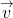in one frame of reference, and that frame of reference is moving with velocity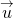past a second reference frame, an observer in the second frame measures the original velocity as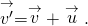This sum of velocities is often referred to as Galilean relativity. If this principle is correct, the pulse of light that the observer measures as traveling with speed c travels at speed c + u measured in the frame of the second observer. If we reasonably assume that the laws of electrodynamics are the same in both frames of reference, then the predicted speed of light (in vacuum) in both frames should be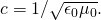Each observer should measure the same speed of the light pulse with respect to that observer’s own rest frame. To reconcile difficulties of this kind, Einstein constructed his special theory of relativity, which introduced radical new ideas about time and space that have since been confirmed experimentally.

### Inertial Frames

All velocities are measured relative to some frame of reference. For example, a car’s motion is measured relative to its starting position on the road it travels on; a projectile’s motion is measured relative to the surface from which it is launched; and a planet’s orbital motion is measured relative to the star it orbits. The frames of reference in which mechanics takes the simplest form are those that are not accelerating. Newton’s first law, the law of inertia, holds exactly in such a frame.

Inertial Reference Frame

An inertial frame of reference is a reference frame in which a body at rest remains at rest and a body in motion moves at a constant speed in a straight line unless acted upon by an outside force.

For example, to a passenger inside a plane flying at constant speed and constant altitude, physics seems to work exactly the same as when the passenger is standing on the surface of Earth. When the plane is taking off, however, matters are somewhat more complicated. In this case, the passenger at rest inside the plane concludes that a net force F on an object is not equal to the product of mass and acceleration, ma. Instead, F is equal to ma plus a fictitious force. This situation is not as simple as in an inertial frame. The term “special” in “special relativity” refers to dealing only with inertial frames of reference. Einstein’s later theory of general relativity deals with all kinds of reference frames, including accelerating, and therefore non-inertial, reference frames.

### Einstein’s First Postulate

Not only are the principles of classical mechanics simplest in inertial frames, but they are the same in all inertial frames. Einstein based the first postulate of his theory on the idea that this is true for all the laws of physics, not merely those in mechanics.

First Postulate of Special Relativity

The laws of physics are the same in all inertial frames of reference.

This postulate denies the existence of a special or preferred inertial frame. The laws of nature do not give us a way to endow any one inertial frame with special properties. For example, we cannot identify any inertial frame as being in a state of “absolute rest.” We can only determine the relative motion of one frame with respect to another.

There is, however, more to this postulate than meets the eye. The laws of physics include only those that satisfy this postulate. We will see that the definitions of energy and momentum must be altered to fit this postulate. Another outcome of this postulate is the famous equation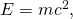which relates energy to mass.

### Einstein’s Second Postulate

The second postulate upon which Einstein based his theory of special relativity deals with the speed of light. Late in the nineteenth century, the major tenets of classical physics were well established. Two of the most important were the laws of electromagnetism and Newton’s laws. Investigations such as Young’s double-slit experiment in the early 1800s had convincingly demonstrated that light is a wave. Maxwell’s equations of electromagnetism implied that electromagnetic waves travel at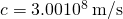in a vacuum, but they do not specify the frame of reference in which light has this speed. Many types of waves were known, and all travelled in some medium. Scientists therefore assumed that some medium carried the light, even in a vacuum, and that light travels at a speed c relative to that medium (often called “the aether”).

Starting in the mid-1880s, the American physicist A.A. Michelson, later aided by E.W. Morley, made a series of direct measurements of the speed of light. They intended to deduce from their data the speed v at which Earth was moving through the mysterious medium for light waves. The speed of light measured on Earth should have been c +v when Earth’s motion was opposite to the medium’s flow at speed u past the Earth, and cv when Earth was moving in the same direction as the medium. The results of their measurements were startling.

Michelson-Morley Experiment

The Michelson-Morley experiment demonstrated that the speed of light in a vacuum is independent of the motion of Earth about the Sun.

The eventual conclusion derived from this result is that light, unlike mechanical waves such as sound, does not need a medium to carry it. Furthermore, the Michelson-Morley results implied that the speed of light c is independent of the motion of the source relative to the observer. That is, everyone observes light to move at speed c regardless of how they move relative to the light source or to one another. For several years, many scientists tried unsuccessfully to explain these results within the framework of Newton’s laws.

In addition, there was a contradiction between the principles of electromagnetism and the assumption made in Newton’s laws about relative velocity. Classically, the velocity of an object in one frame of reference and the velocity of that object in a second frame of reference relative to the first should combine like simple vectors to give the velocity seen in the second frame. If that were correct, then two observers moving at different speeds would see light traveling at different speeds. Imagine what a light wave would look like to a person traveling along with it (in vacuum) at a speed c. If such a motion were possible, then the wave would be stationary relative to the observer. It would have electric and magnetic fields whose strengths varied with position but were constant in time. This is not allowed by Maxwell’s equations. So either Maxwell’s equations are different in different inertial frames, or an object with mass cannot travel at speed c. Einstein concluded that the latter is true: An object with mass cannot travel at speed c. Maxwell’s equations are correct, but Newton’s addition of velocities is not correct for light.

Not until 1905, when Einstein published his first paper on special relativity, was the currently accepted conclusion reached. Based mostly on his analysis that the laws of electricity and magnetism would not allow another speed for light, and only slightly aware of the Michelson-Morley experiment, Einstein detailed his second postulate of special relativity.

Second Postulate of Special Relativity

Light travels in a vacuum with the same speed c in any direction in all inertial frames.

In other words, the speed of light has the same definite speed for any observer, regardless of the relative motion of the source. This deceptively simple and counterintuitive postulate, along with the first postulate, leave all else open for change. Among the changes are the loss of agreement on the time between events, the variation of distance with speed, and the realization that matter and energy can be converted into one another. We describe these concepts in the following sections.

Check Your Understanding Explain how special relativity differs from general relativity.

Special relativity applies only to objects moving at constant velocity, whereas general relativity applies to objects that undergo acceleration.

### Summary

• Relativity is the study of how observers in different reference frames measure the same event.
• Modern relativity is divided into two parts. Special relativity deals with observers in uniform (unaccelerated) motion, whereas general relativity includes accelerated relative motion and gravity. Modern relativity is consistent with all empirical evidence thus far and, in the limit of low velocity and weak gravitation, gives close agreement with the predictions of classical (Galilean) relativity.
• An inertial frame of reference is a reference frame in which a body at rest remains at rest and a body in motion moves at a constant speed in a straight line unless acted upon by an outside force.
• Modern relativity is based on Einstein’s two postulates. The first postulate of special relativity is that the laws of physics are the same in all inertial frames of reference. The second postulate of special relativity is that the speed of light c is the same in all inertial frames of reference, independent of the relative motion of the observer and the light source.
• The Michelson-Morley experiment demonstrated that the speed of light in a vacuum is independent of the motion of Earth about the sun.

### Conceptual Questions

Which of Einstein’s postulates of special relativity includes a concept that does not fit with the ideas of classical physics? Explain.

the second postulate, involving the speed of light; classical physics already included the idea that the laws of mechanics, at least, were the same in all inertial frames, but the velocity of a light pulse was different in different frames moving with respect to each other

Is Earth an inertial frame of reference? Is the sun? Justify your response.

When you are flying in a commercial jet, it may appear to you that the airplane is stationary and Earth is moving beneath you. Is this point of view valid? Discuss briefly.

yes, provided the plane is flying at constant velocity relative to the Earth; in that case, an object with no force acting on it within the plane has no change in velocity relative to the plane and no change in velocity relative to the Earth; both the plane and the ground are inertial frames for describing the motion of the object

### Glossary

first postulate of special relativity
laws of physics are the same in all inertial frames of reference
Galilean relativity
if an observer measures a velocity in one frame of reference, and that frame of reference is moving with a velocity past a second reference frame, an observer in the second frame measures the original velocity as the vector sum of these velocities
inertial frame of reference
reference frame in which a body at rest remains at rest and a body in motion moves at a constant speed in a straight line unless acted on by an outside force
Michelson-Morley experiment
investigation performed in 1887 that showed that the speed of light in a vacuum is the same in all frames of reference from which it is viewed
rest frame
frame of reference in which the observer is at rest
second postulate of special relativity
light travels in a vacuum with the same speed c in any direction in all inertial frames
special theory of relativity
theory that Albert Einstein proposed in 1905 that assumes all the laws of physics have the same form in every inertial frame of reference, and that the speed of light is the same within all inertial frames